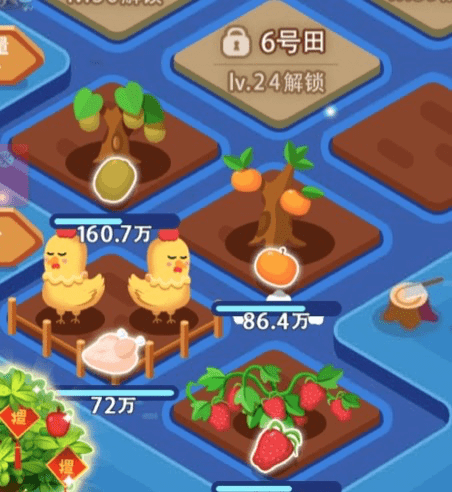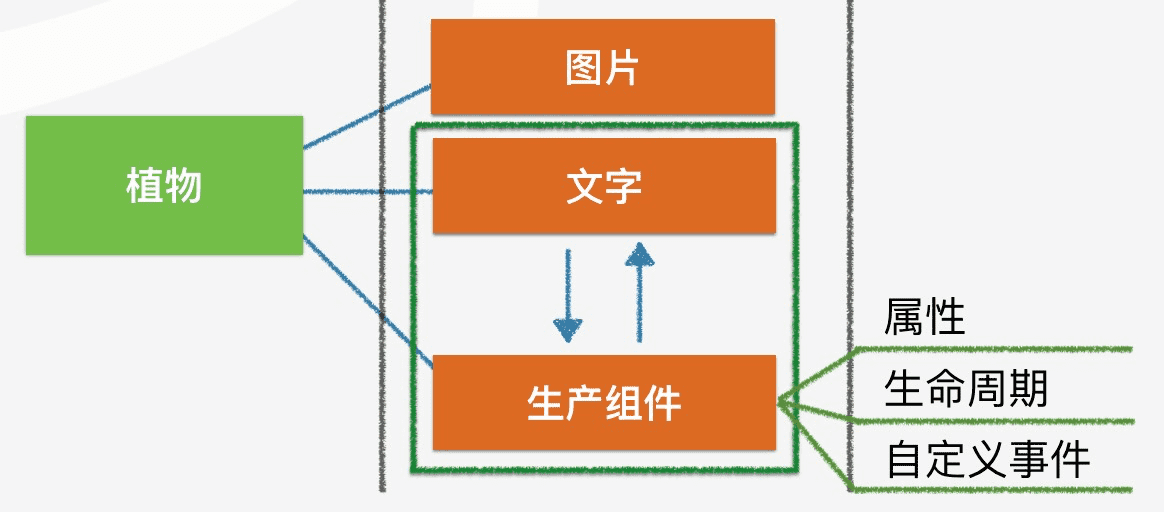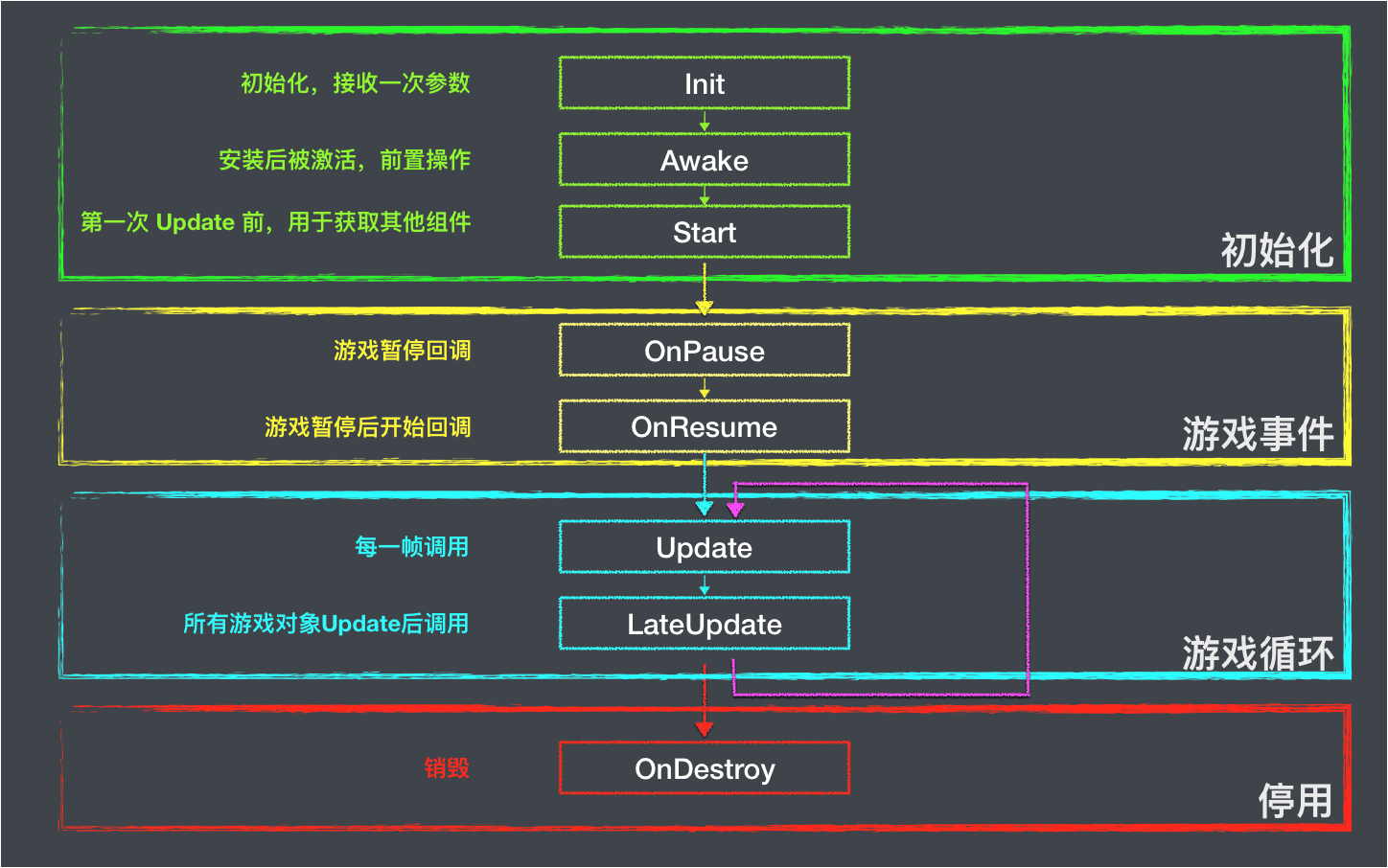# 脚本组件

## 介绍​

### 举例说明：​

Step1: 我们创建一个生产组件 Step2: 在组件上设置描述经验生产的速度，当前经验的属性 Step3: 通过生命周期方法，实时根据生产速度对经验值进行修改，然后修改文字组件上面的文字来展示经验值 Step4: 提供收取作物经验的自定义事件出来，当用户点击植物时调用## 初始化​

``import { Component } from '@eva/eva.js'class Move extends Component {  static componentName = 'Move' // 设置组件的名字}``

## 属性​

``class Move extends Component {  static componentName = 'Move'  speed = {    // 设置属性    // 移动速度    x: 100,    y: 200  }}``

## 组件安装​

``const go = new GameObject('name')go.addComponent(new Move({  speed: {    x: 100,    y: 100  }}))``

## 生命周期​### 初始化​

#### Init​

``interface MoveParams {  speed: {    x: number,    y: number  };}class Move extends Component {  //...  init(params: MoveParams) {    this.speed = params.speed || { x: 0, y: 0 }  }}``

#### Awake​

``class Move extends Component {  // ...  awake() {}}``

#### Start​

``class Move extends Component {  // ...  private img: Img = null    start() {    this.img = this.gameObject.getComponent(Img)  }}``

### 游戏事件​

#### onPause​

`game.pause()`  的时候执行

#### onResume​

`game.resume()`  的时候执行

### 游戏循环​

#### Update​

``class Move extends Component {  // ...  update(e) {    // 让物体按照一定速度移动 位移 = 速度 * 时间    const position = this.gameObject.transform.position    this.gameObject.transform.position.x += this.speed.x * (e.deltaTime / 1000)    this.gameObject.transform.position.y += this.speed.y * (e.deltaTime / 1000)  }}``

## 自定义事件​

``class Move extends Component {  //...  speedUp(value = 20) {    this.speed.x += value    this.speed.y += value  }  speedDown(value = 20) {    this.speed.x -= value    this.speed.y -= value  }}``